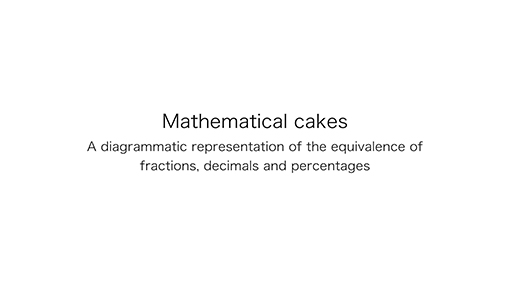Education & Development

### Become an OU studentTeaching mathematics

Start this free course now. Just create an account and sign in. Enrol and complete the course for a free statement of participation or digital badge if available.

# 1.1 Diagrammatic representations of equivalence

The first video shows a mathematical cake which is shaped like a rectangle and has been cut into ten slices. Learners can draw their own mathematical cake on paper. It can have a varied length but it is useful to ensure that the width is 10 cm, which means each slice is 1 cm wide. Diagrams can be very useful aids to teaching concepts in mathematics.

Download this video clip.Video player: Video 1 Mathematical cake: a diagrammatic representation of the equivalence of fractions, decimals and percentagesVideo 1 Mathematical cake: a diagrammatic representation of the equivalence of fractions, decimals and percentages
Interactive feature not available in single page view (see it in standard view).

## Activity 1 Finding equivalences to one-quarter and three-quarters

Timing: Allow 5 minutes

Look at the mathematical cake diagram which was shown in the video. Use it to find equivalences to and .

### Discussion

The mathematical cake diagram can be used to demonstrate the equivalences of percentages, decimals and fractions in tenths.

One-quarter can be found by finding the mid-way point between 0 and one-half. Three quarters would be found mid-way between one-half and one-whole of the cake.

One-quarter is 25% and 0.25.

Three-quarters is 75% and 0.75.